# Analog and Digital Electronics - Online Test

Q1. The output Y of the logic circuit given below isExplaination / Solution: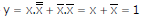Q2.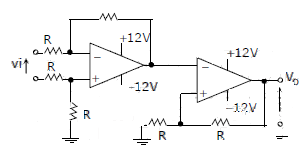The CORRECT transfer characteristic is

Explaination / Solution:

It is a Schmitt trigger and phase shift is zero.

Q3. A low – pass filter with a cut-off frequency of 30Hz is cascaded with a high-pass filter with a cut-off frequency of 20Hz. The resultant system of filters will function as
Explaination / Solution:Q4. The circuit shown is a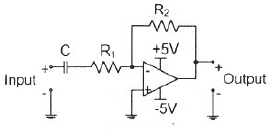Explaination / Solution:Q5. A portion of the main program to call a subroutine SUB in an 8085 environment is given below. : : LXI D,DISP LP : CALL SUB : It is desired that control be returned to LP+DISP+3 when the RET instruction is executed in the subroutine. The set of instructions that precede the RET instruction in the subroutine are
Explaination / Solution:
No Explaination.

Q6. The voltage gain Av of the circuit shown below is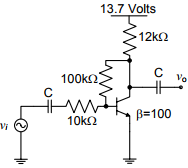Explaination / Solution:Q7. A two – bit counter circuit is shown belowIt the state QAQof the counter at the clock time tn is ‘10’ then the state QAQB of the counter at tn + 3 (after three clock cycles ) will be
Explaination / Solution: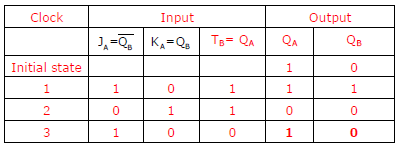Q8. In the circuit shown below what is the output voltage out (Vout) in Volts if a silicon transistor Q and an ideal op-amp are used?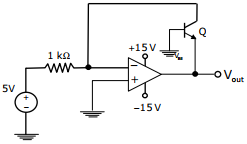Explaination / Solution:
No Explaination.

Q9. In the sum of products function f (X, Y, Z) = ∑(2, 3, 4, 5), the prime implicants are
Explaination / Solution:Q10. In the circuit shown below the op-amps are ideal. Then Vout in Volts is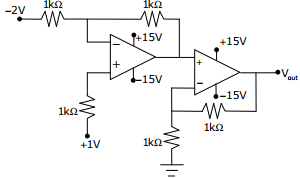Explaination / Solution:
No Explaination.